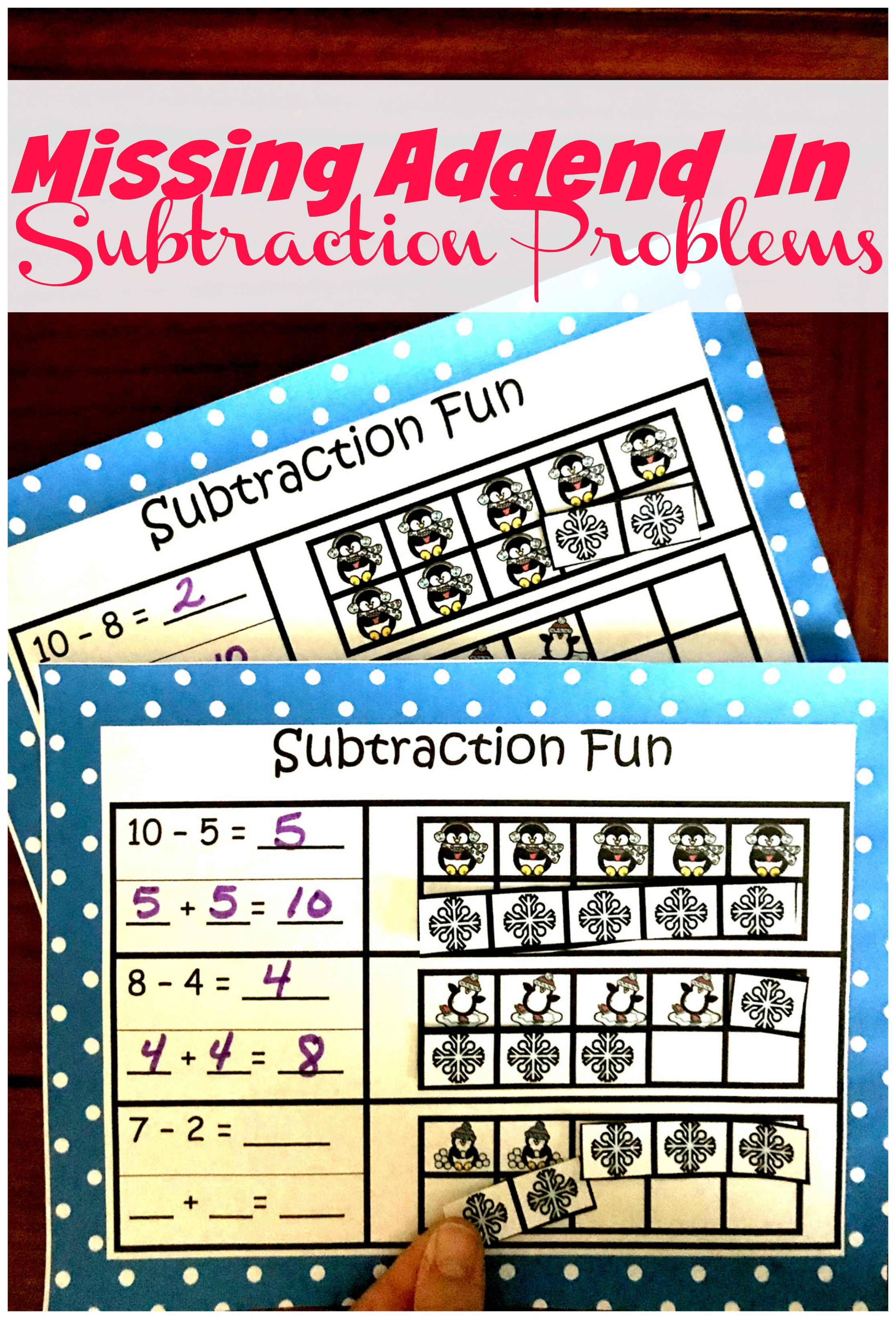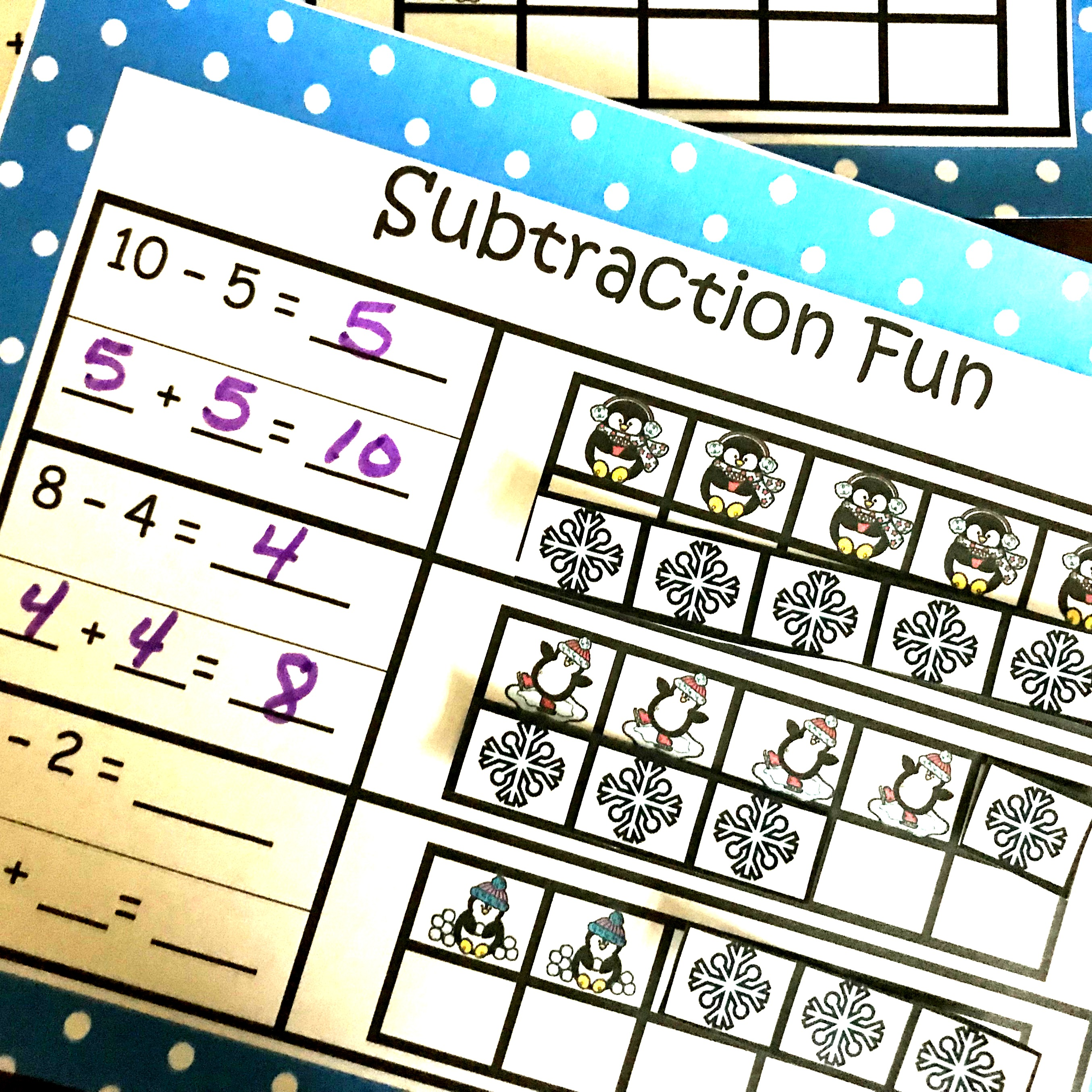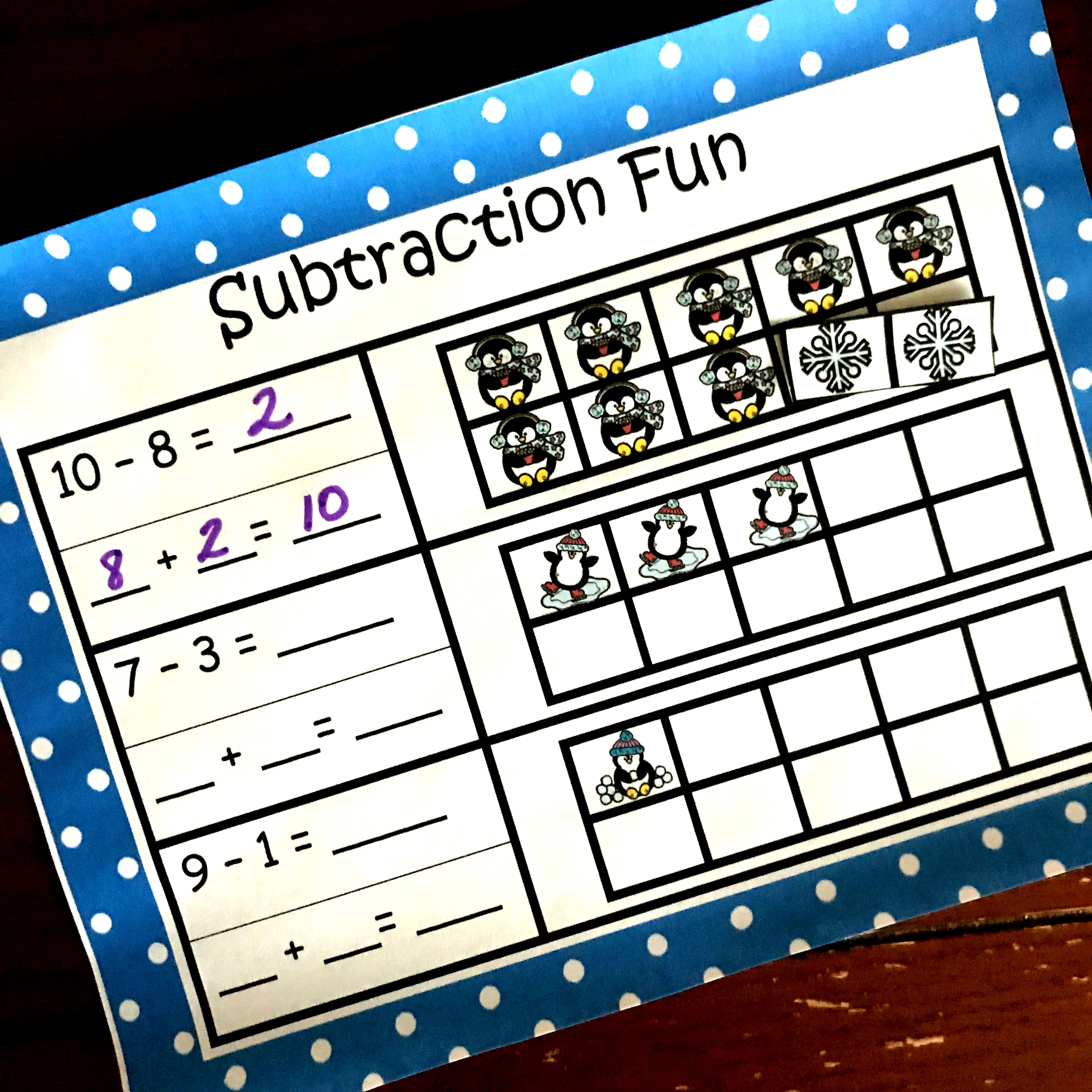Home » Math » Find the Missing Addend in a Subtraction Problem Cut and Paste Activity

Find the Missing Addend in a Subtraction Problem Cut and Paste Activity

Help children understand counting on or missing addend in a subtraction problem with this no-prep cut and paste sheet. They will begin to understand subtraction as an unknown-addend problem. For example, For subtract 12 – 7 by finding the number that makes 12 when added to 7.Math is all about understanding relationships. How do multiplication and division relate? What happens when I add verses subtraction?

And of course, how a missing addend is the same as subtraction. Today’s activity focuses on this concept.

Prep – Work

These are simple no-prep worksheets that will only take seconds to get ready.

1. First, print off the pages you would like your child or children to complete.
2. Next, gather up glue and scissors.

Or you could make this a center that can be used over and over again.

1. Begin by printing of the worksheets and laminating them.
2. Then, cut out the snowflakes and place a magnet on the back of them.
3. Finally, place them worksheets on a cookie sheet and your center is ready to go.

The purpose of this worksheet is to help children see that subtraction can also be a missing addend sentence.

For example, 7 – 3 could also be 3 + ____ = 7.

Example:To begin, the children are given a subtraction expression. Underneath, there are three blanks for them to turn that subtraction expression into an addition expression.

Next, they are given a ten frame already filled with one of the addends. They are to add to the ten frame until they reach the minuend (the first number in a subtraction problem.) Once the ten frame is filled it is easy to see the missing addend.

Talking it out:

We have some sweet little ones that will look at this sheet and get it right away.

They won’t need our help, and this concept will make perfect sense for them.

But we all know, there are others that may need a little more support.

And here is what that conversation may look like.“What is my subtraction problem?”

“10 – 8”

“Very good!!! Now how many of the squares are filled in on the ten frame?

“Eight.”

“That’s Right. Now we are going to count up till we get to the number ten.” (At this point, have the child add pictures until they get to ten.) “How many pictures did you add?

“Two”

“Awesome. Let me see if I got this right. You started with eight and then you added two and now we have ten pictures?”

“Yep.”

“Ok, knowing that can you make an addition sentence for me without using the two?”

8 + ___ = 10

These simple conversations can help children build a greater understanding of how addition and subtraction are connected…..and figure out missing addends in an expression.

You’ve Got This,

Rachel

You may also like:Equation Work